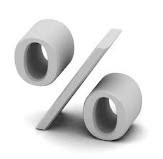## Tuesday, 8 May 2012

### Nota Ringkas (Perpuluhan dan Peratusan)-Bab 4 dan Bab 5-

nota ringkas ini sy ambil daripada laman web

smoga sama2 kita memahaminya :)

Fikir2kan & Selamat berjuang ("-")v

Perpuluhan VS PeratusChapter 4 :DECIMALS

4.1Decimals and Fractions
1.decimal is a fraction with adenominator of 10 or a power of 10.2.To convert a fraction into a decimal,divide the numerator by thedenominator.3.To change a decimal into a fraction.Divide it by the power of 10 such as 10,100, 1 000,..Example:i)4.052
=
0.4 is read as zero point four ii)1008308.3
+=
3.08 is read as three point zero eight
4.2Place Value and Digit Value
1.TensunitsDecimal pointtenthshundredths12.4512.45 = 10 + 2 + 0.4 +0.05= 10 + 2 +1005104
+
2.The decimal places are the placesoccupied by the digit after the decimal point.Example:i) 7.9
1 digit to the right of the decimal  point
Therefore, 7.9 has 1 decimal point.ii) 7.94
2
digits to the right of the decimal pointTherefore, 7.94 has 2 decimal points.iii) 7.948
3 digits to the right of the decimal  point
Therefore, 7.948 has 3 decimal points.
4.3Addition and Subtraction oDecimals
To add or subtract decimals, arrange thedigits of the same place value in the samevertical column.Example:27.064 +1.3 0.91 29.27427 . 0641. 39129 . 274
4.4Multiplication and Division oDecimals
To multiply decimals(a) Multiply the decimals in the same way aswhole numbers.(b) The number of decimal places in theanswer is equal to the sum of decimal places in the individual decimals.Example :i)3.41.5.8653.45 d.p.)1.7 d.p.)24153455.865 d.p.)ii)6.÷ 0.0=369003.09.6
=
= 230
4.5CombineOperations
In performing the combined operations of decimals, we follow the same rule asthose for whole nuir (a) Work out the expressions in brackets.(b) Do multiplication and division from leftto right.(c) Do addition and subtraction from left toright.Example:6x ( 4.1 – 2.12 ) ÷ 0.81.98 ÷ 0.811.88 / 0.8= 14.85
Chapter 5 PERCENTAGES (%)5.1 Percentages
1.
Percentage is a fraction out of 100.
2.
Change a fraction into a percentage,
multiply it by 100%.
Example:
43
x 100% = 75%3.To change a decimal into a percentage,
multiply by 100%.
Example: 0.29 x 100% = 29%4.To change a percentage into fraction,
divide by 100% and reduce to its lowest terms.
Example:1.30% =
100301
+
=
1031
5.To change a percentage into a decimal,
divide by 100%.
Example: 16% =
10016
= 0.16
5.2
Perform computations
1.
To find the percentage of a quantity,
change into fraction and multiply by thequantity.
Example: 30% of 400=
10030
x 400= 120
2.
To find the percentage one number is of another,
change both quantities to the same unit, form a fraction and multiplyby 100%
Exampleo10=
103
x 100%= 30%
3.
To find a number given the percentage,
first find the value of 1 % then find the value of 100% of thenumber.
Example:Given 10% of a number = 501% of a number 50 =
1050
The number =
1050
x 100= 500
4.To find the percentage increase or decrease 5.
Increase
may be dividend, commission,simple interest or profit.Example :300 is increased by 20 %Value of increase =30010020
x
= 60Final value = 300 +60= 3606.
Decrease
may be discount or loss.Example:Selling price = RM 500Discoun30%Decrease =50010030
xRM
= RM 150Selling price = 500 – 150=RM 350Percentage change =change in value 100%original value

Increase= % increase x original valueFinal value= Original value + increaseDecrease= % decrease x original valueFinal value= Original value - decrease